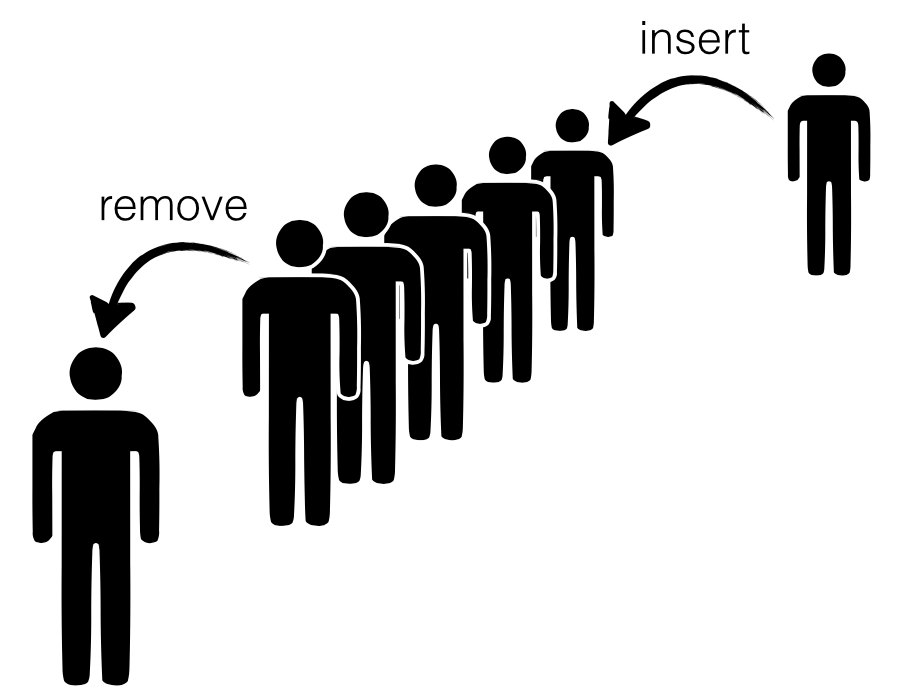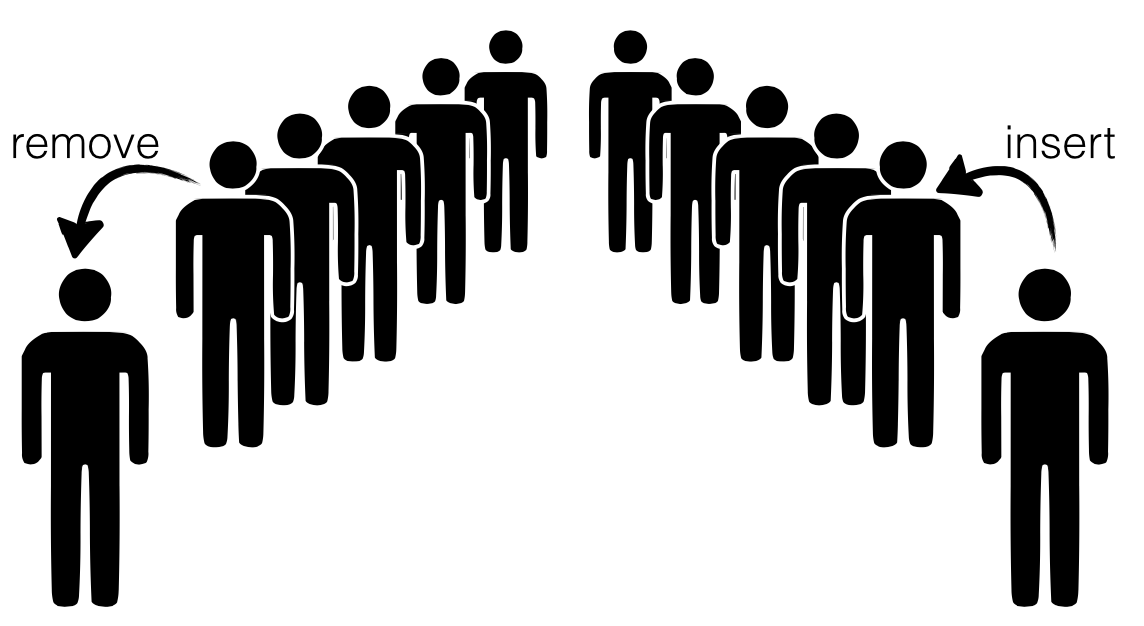# Case Study: Okasaki’s Lazy Queues

Lets start with a case study that is simple enough to explain without pages of code, yet complex enough to show off whats cool about dependency: Chris Okasaki’s beautiful Lazy Queues. This structure leans heavily on an invariant to provide fast insertion and deletion. Let’s see how to enforce that invariant with LiquidHaskell.

## Queues

A queue is a structure into which we can insert and remove data such that the order in which the data is removed is the same as the order in which it was inserted.Figure 1.1: A Queue is a structure into which we can insert and remove elements. The order in which the elements are removed is the same as the order in which they were inserted.

To efficiently implement a queue we need to have rapid access to both the front as well as the back because we remove elements from former and insert elements into the latter. This is quite straightforward with explicit pointers and mutation – one uses an old school linked list and maintains pointers to the head and the tail. But can we implement the structure efficiently without having stoop so low?

Chris Okasaki came up with a very cunning way to implement queues using a pair of lists – let’s call them front and back which represent the corresponding parts of the Queue.

• To insert elements, we just cons them onto the back list,
• To remove elements, we just un-cons them from the front list.Figure 1.2: We can implement a Queue with a pair of lists; respectively representing the front and back.

The catch is that we need to shunt elements from the back to the front every so often, e.g. we can transfer the elements from the back to the front, when:

1. a remove call is triggered, and
2. the front list is empty.Figure 1.3: Transferring Elements from back to front.

Okasaki's first insight was to note that every element is only moved once from the back to the front; hence, the time for insert and remove could be O(1) when amortized over all the operations. This is perfect, except that some set of unlucky remove calls (which occur when the front is empty) are stuck paying the bill. They have a rather high latency up to O(n) where n is the total number of operations.

Okasaki's second insight saves the day: he observed that all we need to do is to enforce a simple balance invariant:

$\mbox{Size of front} \geq \mbox{Size of back}$

If the lists are lazy i.e. only constructed as the head value is demanded, then a single remove needs only a tiny O(log n) in the worst case, and so no single remove is stuck paying the bill.

Lets implement Queues and ensure the crucial invariant(s) with LiquidHaskell. What we need are the following ingredients:

1. A type for Lists, and a way to track their size,

2. A type for Queues which encodes the balance invariant

3. A way to implement the insert, remove and transfer operations.

## Sized Lists

The first part is super easy. Let’s define a type:

data SList a = SL { size :: Int, elems :: [a] }

We have a special field that saves the size because otherwise, we have a linear time computation that wrecks Okasaki’s careful analysis. (Actually, he presents a variant which does not require saving the size as well, but that’s for another day.)

How can we be sure that size is indeed the real size of elems? Let’s write a function to measure the real size:

{-@ measure realSize @-} realSize :: [a] -> Int realSize [] = 0 realSize (_:xs) = 1 + realSize xs

Now, we can simply specify a refined type for SList that ensures that the real size is saved in the size field:

{-@ data SList a = SL { size :: Nat , elems :: {v:[a] | realSize v = size} } @-}

As a sanity check, consider this:

okList = SL 1 ["cat"] -- accepted badList = SL 1 [] -- rejected

Lets define an alias for lists of a given size N:

{-@ type SListN a N = {v:SList a | size v = N} @-}

Finally, we can define a basic API for SList.

To Construct lists, we use nil and cons:

{-@ nil :: SListN a 0 @-} nil = SL 0 [] {-@ cons :: a -> xs:SList a -> SListN a {size xs + 1} @-} cons x (SL n xs) = SL (n+1) (x:xs)

Exercise: (Destructing Lists): We can destruct lists by writing a hd and tl function as shown below. Fix the specification or implementation such that the definitions typecheck.

{-@ tl :: xs:SList a -> SListN a {size xs - 1} @-} tl (SL n (_:xs)) = SL (n-1) xs tl _ = die "empty SList" {-@ hd :: xs:SList a -> a @-} hd (SL _ (x:_)) = x hd _ = die "empty SList"

Hint: When you are done, okHd should be verified, but badHd should be rejected.

{-@ okList :: SListN String 1 @-} okHd = hd okList -- accepted badHd = hd (tl okList) -- rejected

## Queue Type

It is quite straightforward to define the Queue type, as a pair of lists, front and back, such that the latter is always smaller than the former:

{-@ data Queue a = Q { front :: SList a , back :: SListLE a (size front) } @-} data Queue a = Q { front :: SList a , back :: SList a }

The alias SListLE a L corresponds to lists with at most N elements:

{-@ type SListLE a N = {v:SList a | size v <= N} @-}

As a quick check, notice that we cannot represent illegal Queues:

okQ = Q okList nil -- accepted, |front| > |back| badQ = Q nil okList -- rejected, |front| < |back|

## Queue Operations

Almost there! Now all that remains is to define the Queue API. The code below is more or less identical to Okasaki’s (I prefer front and back to his left and right.)

The Empty Queue is simply one where both front and back are both empty:

emp = Q nil nil

To Remove an element we pop it off the front by using hd and tl. Notice that the remove is only called on non-empty Queues, which together with the key balance invariant, ensures that the calls to hd and tl are safe.

remove (Q f b) = (hd f, makeq (tl f) b)

Exercise: (Whither pattern matching?): Can you explain why we (or Okasaki) didn’t use pattern matching here, and have instead opted for the explicit hd and tl?

Exercise: (Queue Sizes): If you did the List Destructing exercise above, then you will notice that the code for remove has a type error: namely, the calls to hd and tl may fail if the f list is empty.

1. Write a measure to describe the queue size,
2. Use it to complete the definition of QueueN below, and
3. Use it to give remove a type that verifies the safety of the calls made to hd and tl.

Hint: When you are done, okRemove should be accepted, badRemove should be rejected, and emp should have the type shown below:

-- | Queues of size N {-@ type QueueN a N = {v:Queue a | true} @-} okRemove = remove example2Q -- accept badRemove = remove example0Q -- reject {-@ emp :: QueueN _ 0 @-} {-@ example2Q :: QueueN _ 2 @-} example2Q = Q (1 cons (2 cons nil)) nil {-@ example0Q :: QueueN _ 0 @-} example0Q = Q nil nil

To Insert an element we just cons it to the back list, and call the smart constructor makeq to ensure that the balance invariant holds:

insert e (Q f b) = makeq f (e cons b)

Exercise: (Insert): Write down a type for insert such that replicate and okReplicate are accepted by LiquidHaskell, but badReplicate is rejected.

{-@ replicate :: n:Nat -> a -> QueueN a n @-} replicate 0 _ = emp replicate n x = insert x (replicate (n-1) x) {-@ okReplicate :: QueueN _ 3 @-} okReplicate = replicate 3 "Yeah!" -- accept {-@ badReplicate :: QueueN _ 3 @-} badReplicate = replicate 1 "No!" -- reject

To Ensure the Invariant we use the smart constructor makeq, which is where the heavy lifting happens. The constructor takes two lists, the front f and back b and if they are balanced, directly returns the Queue, and otherwise transfers the elements from b over using the rotate function rot described next.

{-@ makeq :: f:SList a -> b:SList a -> QueueN a {size f + size b} @-} makeq f b | size b <= size f = Q f b | otherwise = Q (rot f b nil) nil

Exercise: (Rotate): The Rotate function rot is only called when the back is one larger than the front (we never let things drift beyond that). It is arranged so that it the hd is built up fast, before the entire computation finishes; which, combined with laziness provides the efficient worst-case guarantee. Write down a type for rot so that it typechecks and verifies the type for makeq.

Hint: You may have to modify a precondition in makeq to capture the relationship between f and b.

rot f b acc | size f == 0 = hd b cons acc | otherwise = hd f cons rot (tl f) (tl b) (hd b cons acc)

Exercise: (Transfer): Write down a signature for take which extracts n elements from its input q and puts them into a new output Queue. When you are done, okTake should be accepted, but badTake should be rejected.

take :: Int -> Queue a -> (Queue a, Queue a) take 0 q = (emp , q) take n q = (insert x out , q'') where (x , q') = remove q (out, q'') = take (n-1) q' {-@ okTake :: (QueueN _ 2, QueueN _ 1) @-} okTake = take 2 exampleQ -- accept badTake = take 10 exampleQ -- reject exampleQ = insert "nal" $insert "bob"$ insert "alice" \$ emp

## Recap

Well there you have it; Okasaki’s beautiful lazy Queue, with the invariants easily expressed and checked with LiquidHaskell. This example is particularly interesting because

1. The refinements express invariants that are critical for efficiency,
2. The code introspects on the size to guarantee the invariants, and
3. The code is quite simple and we hope, easy to follow!